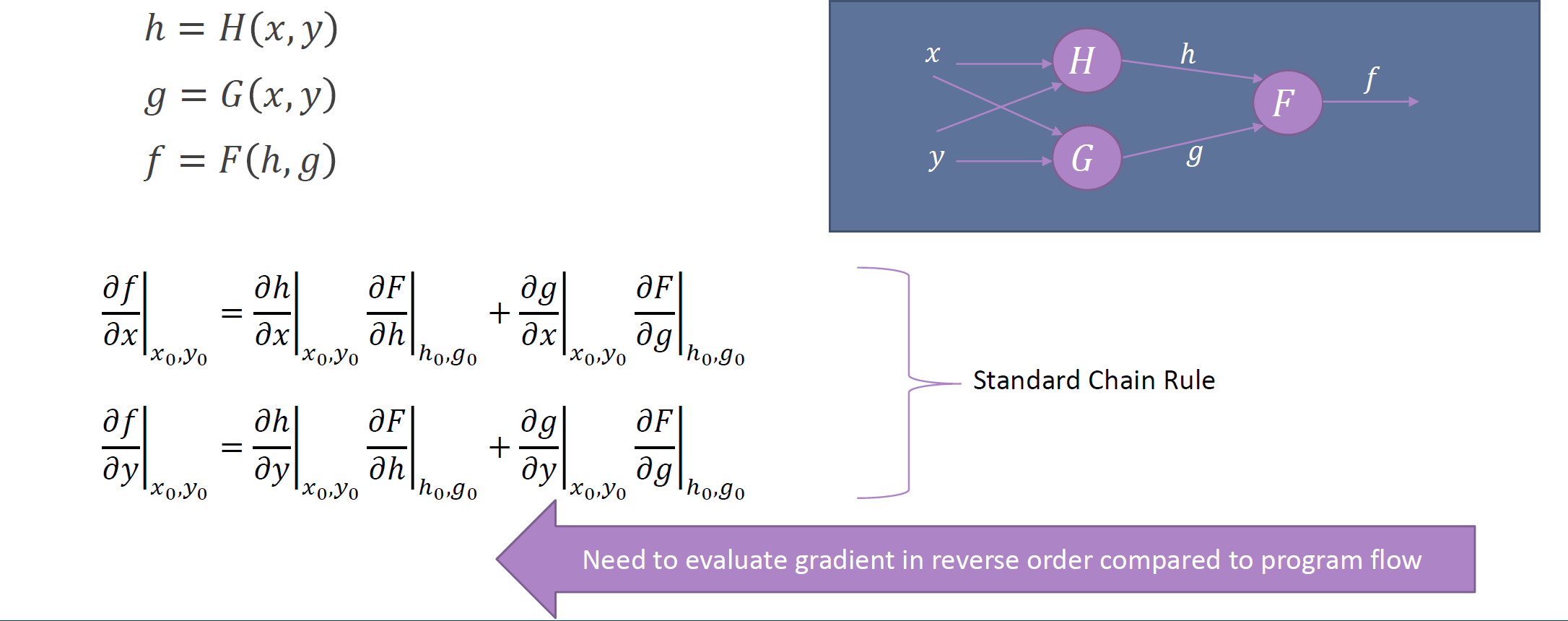Understanding what a PyTorch leaf node is intuitive when you remember that PyTorch automatic differentiation is in the backward direction compared to the way the program is executed to compute its value – i.e., it is a backward-mode automatic differentiation.

Below are a couple of slides I’ve used before to illustrate backward mode autodiff:In PyTorch leaf nodes are therefore the values from which the computation begins. Here a simple program illustrating this:

``````
# The following two values are the leaf nodes

# The remaining nodes are not leaves:
def H(z1, z2):
return T.sin(z1**3)*T.cos(z2**2)

def G(z1, z2):
return T.exp(z1)+T.log(z2)

def F(z1, z2):
return z1**3*z2**0.5

h=H(x,y)
g=G(x,y)
f=F(h,g)
r=(f).sum()
``````

The graph illustrating this is below – the leaf nodes are the two blue nodes (i.e., our x and y above) at the start of the computational graph: